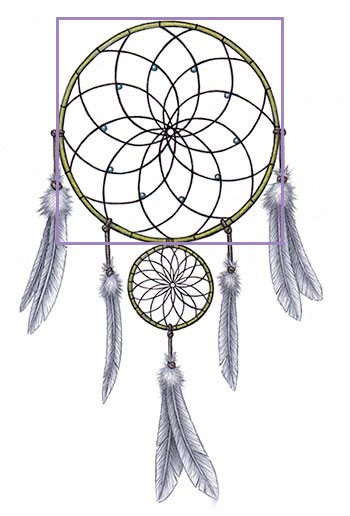SEARCH HOMEMath Central Quandaries & Queries$Question from Faye: I have been asked to build a dreamcatcher, which is round into a 3x3foot area. What would the circumference be that I would need to make the hoop? I am thinking it is 9.42 feet long but am not good in math.Hi Faye, I took an image of a dreamcatcher from the Chrystallinks site and put a square frame around the large circle.If the frame is a 3 foot square as in your situation then you can see by the image that the diameter of the circle is 3 feet. The circumference of a circle is$\pi \; d$feet where$d$is the diameter in feet and$\pi$is approximately 3.1416. Thus in your situation the circumference of the circle or hoop is$\pi \times 3 = 9.42\$ feet.